Tamilnadu State Board New Syllabus Samacheer Kalvi 11th Maths Guide Pdf Chapter 6 Two Dimensional Analytical Geometry Ex 6.3 Text Book Back Questions and Answers, Notes.

## Tamilnadu Samacheer Kalvi 11th Maths Solutions Chapter 6 Two Dimensional Analytical Geometry Ex 6.3

Question 1.
Show that the lines 3x + 2y + 9 = 0 and 12x + 8y – 15 = 0 are parallel lines.
The equations of the given lines are
3x + 2y + 9 = 0 ——- (1)
12x + 8y – 15 = 0 ——- (2)m1 = m2
∴ The given lines are parallel.Question 2.
Find the equation of the straight line parallel to 5x – 4y + 3 = 0 and having x – intercept 3.
The equation of any line parallel to 5x – 4y + 3 = 0 is
5x – 4y + k = 0 ……….. (1)
The x – intercept of line (I) is obtained by putting
y = 0 in the equation.
(1) ⇒ 5x – 4(0) + k = 0
5x = – k ⇒ x = $$-\frac{k}{5}$$
Given that the x – intercept in 3
∴ $$-\frac{k}{5}$$ = 3 ⇒ k = – 15
∴ The equation of the required line is
5x – 4y – 15 = 0

Question 3.
Find the distance between the line 4x + 3y + 4 = 0 and a point
(i) (- 2, 4)
(ii) (7, – 3)
(i) The distance between the line ax + by + c = 0 and the point (x1, y1) is d = $$\frac{a x_{1}+b y_{1}+c}{\sqrt{a^{2}+b^{2}}}$$
Here (x1, y1) = (- 2, 4) and the equation of the line is 4x + 3y + 4 = 0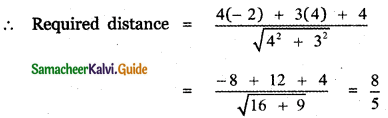(ii) Given point (x1, y1) = (7, – 3)
Given line 4x + 3y + 4 = 0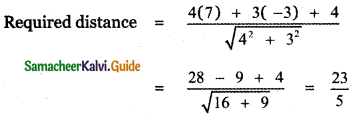Question 4.
Write the equation of the lines through the point (1, – 1)
(i) Parallel to x + 3y – 4 = 0
(ii) Perpendicular to 3x + 4y = 6
(i) Any line parallel to x + 3y – 4 = 0 will be of the form x + 3y + k = 0.
It passes through (1,-1) ⇒ 1 – 3 + k = 0 ⇒ k = 2
So the required line is x + 3y + 2 = 0

(ii) Any line perpendicular to 3x + 4y – 6 = 0 will be of the form 4x – 3y + k = 0.
It passes through (1, -1) ⇒ 4 + 3 + k = 0 ⇒ k = -7.
So the required line is 4x – 3y – 7 = 0

Question 5.
If (- 4, 7 ) is one vertex of a rhombus and if the equation of one diagonal is 5x – y + 7 = 0 then find the equation of another diagonal.In a rhombus, the diagonal cut at right angles.
The given diagonal is 5x – y + 7 = 0 and (- 4, 7) is not a point on the diagonal.
So it will be a point on the other diagonal which is perpendicular to 5x – y + 7 = 0.
The equation of a line perpendicular to 5x – y + 7 = 0 will be of the form x + 5y + k = 0.
It passes through (-4, 7) ⇒ -4 + 5(7) + k = 0 ⇒ k = -31
So the equation of the other diagonal is x + 5y – 31 = 0Question 6.
Find the equation of the lines passing through the point of intersection of the lines 4x – y + 3 = 0 and 5x + 2y + 7 = 0 and
(i) through the point (-1,2)
(ii) parallel to x – y + 5 =0
(iii) perpendicular to x – 2y + 1 =0
The equation of the straight line passing through the point of intersection of the lines.
4x – y + 3 = 0 and 5x + 2y + 7 = 0 is
( 4x – y + 3) + λ (5x + 2y + 7) = 0 ……… (1)

(i) Through the point (-1,2)
Given that line(1) passes through the point (-1, 2)
(1) ⇒ (4(-1) – 2 + 3) + λ (5(- 1) + 2(2) + 7) = 0
(-4 – 2 + 3) + λ (-5 + 4 + 7) = 0
– 3 + 6 λ = 0 ⇒ λ = $$\frac{3}{6}$$ = $$\frac{1}{2}$$
∴ The equation of the required line is
(4x – y + 3) + $$\frac{1}{2}$$ (5x + 2y + 7) = 0
2(4x – y + 3) + (5x + 2y + 7) = 0
8x – 2y + 6 + 5x + 2y + 7 = 0
13x +13 = 0 ⇒ x + 1 = 0

(ii) Equation of a line parallel to x – y + 5 = 0 will be of the form x – y + k = 0.
It passes through (-1, -1) ⇒ -1 + 1 + k = 0 ⇒ k = 0.
So the required line is x – y = 0 ⇒ x = y.

(iii) Perpendicular to x – 2y + 1 = 0
Given that the line (1) perpendicular to the line
x – 2y + 1 = 0 ………….. (3)
(1) ⇒ (4x – y + 3) + λ (5x + 2y + 7) = 0
4x – y + 3 + 5λx + 2λy + 7λ = 0
(4 + 5λ)x + (2λ – 1 )y + (3 + 7λ) = 0 ……….. (4)
Slope of this line (3) = $$-\frac{4+5 \lambda}{2 \lambda-1}$$
Slope of line (2) = $$-\frac{1}{-2}=\frac{1}{2}$$
Given that line (3) and line (4) are perpendicular4 + 5λ = 2(2λ – 1 )
4 + 5λ = 4λ – 2
λ = – 6
Substituting the value of λ in equation (1) we have
(4x – y + 3) – 6 (5x + 2y + 7) = 0
4x – y + 3 – 30x – 12y – 42 = 0
-26x – 13y – 39 =0
2x + y + 3 = 0
which is the required equation.Question 7.
Find the equations of two straight line which are parallel to the line 12x + 5y + 2 = 0 and at a unit distance from the point (1, – 1).
The equation of the given line is
12x + 5y + 2 = 0 ……… (1)
Equation of any line parallel to the line (1) is
12x + 5y + k = 0 ………. (2)
Given that line (2) is at a unit distance from the point (1, – 1)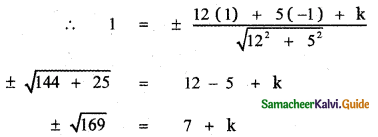k = – 7 ± 13
k = -7 + 13 or k = – 7 – 13
k = 6 or k = – 20
∴ The equation of the required lines are
12x + 5y + 6 = 0 and 12x + 5y – 20 = 0

Question 8.
Find the equations of straight lines which are perpendicular to the line 3x + 4y – 6 = 0 and are at a distance of 4 units from (2, 1).
The equation of the given line is
3x + 4y – 6 = 0 …………. (1)
The equation of any line perpendicular to line (1) is
4x – 3y + k = 0 …………. (2)
Given that this line is 4 units from the point (2, 1)± 4 = $$\frac{5+k}{5}$$
5 + k = ± 20
k = ± 20 – 5
k = 20 – 5 or k = -20
k = 15 or k = -25
∴ The equation of the required lines are
4x – 3y + 15 = 0 and 4x – 3y – 25 = 0

Question 9.
Find the equation of a straight line parallel to 2x + 3y = 10 and which is such that the sum of its intercepts on the axes is 15.
The equation of the given line is
2x + 3y = 10 ………….. (1)
The equation of any line parallel to (1) is
2x + 3y = k …………. (2)Given that the sum of the intercepts of the line (2) on the axes is 15∴ The equation of the required line is 2x + 3y = 18Question 10.
Find the length of the perpendicular and the coordinates of the foot of the perpendicular from (-10, -2) to the line x + y – 2 = 0.
The coordinate of the foot of the perpendicular from the point (x1, y1) on the line ax + by + c = 0 is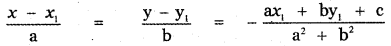∴ The coordinate of the foot of the perpendicular from the point (- 10, – 2) on the line x + y – 2 = 0 is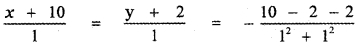x + 10 = y + 2 = $$\frac{14}{2}$$
x + 10 = y + 2 = 7
x + 10 = 7, y + 2 = 7
x = – 3, y = 5
∴ The required foot of the perpendicular is (- 3, 5).
Length of the perpendicular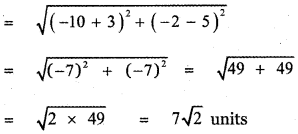Question 11.
If p1 and p2 are the lengths of the perpendiculars from the origin to the straight lines x sec θ + y cosec θ = 2a and x cos θ – y sin θ = a cos 2θ, then prove that p12 + p22 = a2.
Given P1 is the length of the perpendicular from the origin to the straight line
x sec θ + y cosec θ – 2a = 0Also given P2 is the length of the perpendicular from the origin to the straight line
x cos θ – y sin θ – a cos 2θ = 0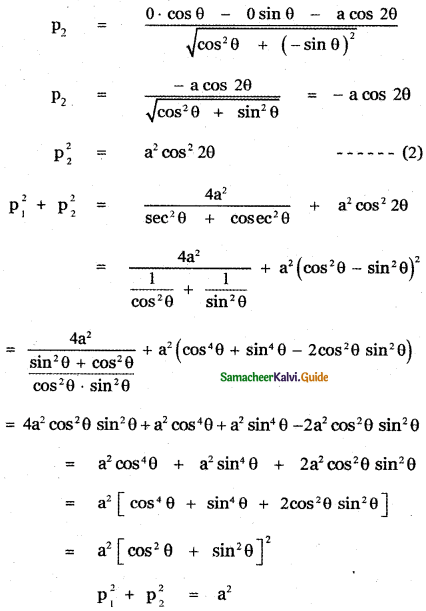Question 12.
Find the distance between the parallel lines
(i) 12x + 5y = 7 and 12x + 5y + 7 = 0
(ii) 3x – 4y + 5 = 0 and 6x – 8y – 15 = 0
(i) 12x + 5y = 7 and 12x + 5y + 7 = 0
The equation of the given lines are
12x + 5y – 7 = 0 ……….. (1)
12x + 5y + 7 = 0 ……….. (2)
The distance between the parallel lines
ax + by + c1 = 0 and ax + by + c2 = 0 is
The equation of any line parallel to (1) isThe distance cannot be negative
∴ Required distance = $$\frac{14}{13}$$

(ii) 3x – 4y + 5 = 0 and 6x – 8y -15 = 0
The equation of the given lines are
3x – 4y + 5 = 0 ……….. (1)
6x – 8y – 15 = 0
3x – 4y – 1 = 0 ………… (2)
The distance between the parallel lines (1) and (2) is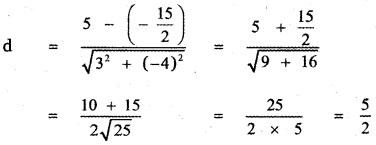Question 13.
Find the family of straight lines
(i) Perpendicular
(ii) Parallel to 3x + 4y – 12
(i) Equation of lines perpendicular to 3x + 4y – 12 = 0 will be of the form 4x – 3y + k = 0, k ∈ R
(ii) Equation of lines parallel to 3x + 4y – 12 = 0 will be of the form 3x + 4y + k = 0, k ∈ RQuestion 14.
If the line joining two points A (2, 0) and B (3, 1) is rotated about A in an anticlockwise direction through an angle of 15° , then find the equation of the line in the new position.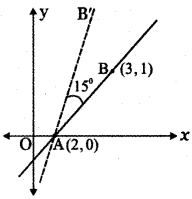Slope of the line AB
m = tan θ = $$\frac{1-0}{3-2}$$
tan θ = 1
θ = 45°
∴ The line AB makes an angle 45° with x-axis.
Given that the line AB is rotated through an angle of 15° about the point A in the anticlockwise direction.
∴ The angle made by the new line AB’ is 45° + 15° = 60°
Slope of the new line AB’ is m1 = tan 60° = √3
∴ The equation of the new line AB’ is the equation of the straight line passing through the point A (2, 0) and having slope m1 = √3
y – 0 = √3 (x – 2)
y = √3x – 2√3
√3x – y – 2√3 = 0

Question 15.
A ray of light coming from the point (1, 2) is reflected at a point A on the x – axis and it passes through the point (5, 3). Find the coordinates of point A.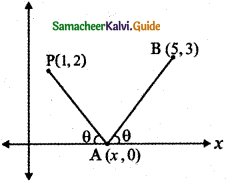Let P(1, 2) and (5, 3) are the given points.
By the property of reflection,
∠XAB = ∠OAP = θ
(Angle of incidence = Angle of reflection)
Slope of the line OA (x – axis) m1 = 0
Slope of the line joining the points P (1, 2) and A (x, 0)
Slope of AP, m2 = $$\frac{2-0}{1-x}=\frac{2}{1-x}$$
Slope of the line joining the points B (5, 3 ) and A (x, 0)
Slope of AP, m3 = $$\frac{3-0}{5-x}=\frac{3}{5-x}$$From equations (1) and (2)
$$\frac{3}{5-x}$$ = –$$\frac{2}{1-x}$$
3(1 – x) = – 2 (5 – x)
3 – 3x = – 10 + 2x
2x + 3x = 10 + 3
5x = 13 ⇒ x = $$\frac{13}{5}$$
∴ The required point A is $$\left(\frac{13}{5}, 0\right)$$Question 16.
A line is drawn perpendicular to 5x = y + 7. Find the equation of the line if the area of the triangle formed by this line with co-ordinate axes is 10. sq. units.Let the given line be PQ whose equation is
5x = y + 7
5x – y – 7 = 0 ——- (1)
Let AB be the line perpendicular to the line PQ such that the area of the triangle OAB is 10 units.
The equation of the line AB is
– x – 5y + k = 0
x + 5y – k = 0
x + 5y = k ——- (2)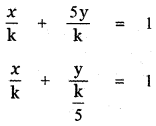∴ A is (k, 0) and B is (0, $$\frac{\mathrm{k}}{5}$$)
OA = k and OB = $$\frac{\mathrm{k}}{5}$$
Area of ∆OAB = $$\frac{1}{2}$$ × OA × OB
= $$\frac{1}{2}$$ × k × $$\frac{\mathrm{k}}{5}$$
Given area of ∆ OAB = 10
∴ $$\frac{\mathrm{k}^{2}}{10}$$ = 10
k2 = 100 ⇒ k = ±10
∴ The required equation of the straight line is
x + 5y = ±10

Question 17.
Find the image of the point (- 2, 3) about the line x + 2y – 9 = 0.
The image of the point (x1, y1) about the line ax + by + c = 0 is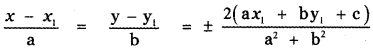∴ The image of the point (- 2, 3) about the line x + 2y – 9 = 0 is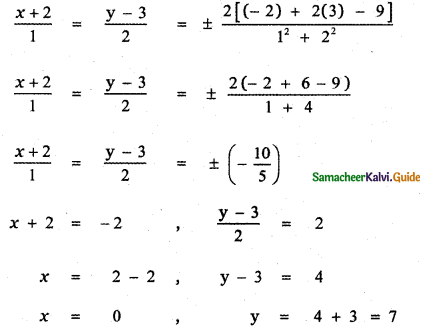∴ The required point is (0, 7)Question 18.
A photocopy store charges ₹ 1.50 per copy for the first 10 copies and ₹ 1.00 per copy after the 10th copy. Let x be the number of copies, and y be the total cost of photo coping.
(i) Draw graph the cost as x goes from 0 to 50 copies
(ii) Find the cost of making 40 copies.
(i) Draw graph of the cost as x goes from 0 to 50 copies:
Let x represent the number of copies and y represent the cost of photocopying.
Given photo copying charge for 1 copy is Rs. 1.50 for the first 10 copies and Rs. 1.00 per copy after the 10th copy.
∴ The relation connecting the number of copies and cost of photocopying charge is given by
y = 1.50x, 0 ≤ x ≤ 10
y = 10(1.50) + (x – 10) (1)
y = 15 + x – 10
y = x + 5 ………… (1)The graph for 0 to 50 copies: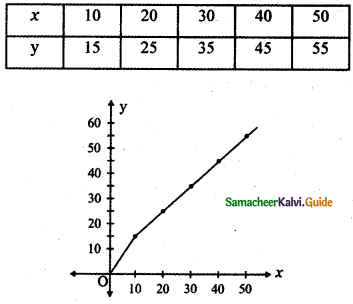When x = 10, y = 1.50 × x
⇒ y = 1.50 × 10 = 15
The corresponding point is (10 , 15)
When x = 20, y = x + 5
⇒ y = 20 + 5 = 25
The corresponding point is (20 , 25)
When x = 30, y = x + 5
⇒ y = 30 + 5 = 35
The corresponding point is (30, 35)
When x = 40, y = 40 + 5 = 45
The corresponding point is (40, 45 )
When x = 50, y = 50 + 5 = 55
The corresponding point is (50, 55 )
The cost of 40 copies is the value of y
When x = 40 , y = 40 + 5 = 45 rupees
Cost of 40 copies = 45 rupeesQuestion 19.
Find at least two equations of the straight lines in the family of file lines y = 5x + b for which b and the x – coordinate of the point of intersection of the lines with 3x – 4y = 6 are integers.
The equations of the given straight lines are
y = 5x + b ……….. (1)
3x – 4y = 6 ……….. (2)
To find atleast two equations from the family y = 5x + b for which b is an integer and x – coordinate of the point of intersection of (1) and (2) is an integer. Solving (1)and (2) using equation (1) inequation (2) (2) ⇒ 3x – 4 (5x + b) = 6
3x – 20x – 4b = 6
-17x = 6 + 4bThe corresponding equation of the line is = 5x + 7
When b = – 10, we have x = $$\frac{6-40}{-17}$$
= $$\frac{-34}{-17}$$ = 2
The corresponding equation of the line is y = 5x – 10
Thus y = 5x + 7 and y = 5x – 10 are the two straight lines belonging to the family such that b is an integer and the x – coordinate of the point of intersection with the line (2) is an integer.

Question 20.
Find all the equations of the straight lines in the family of the lines y = mx – 3 for which m and the x – coordinate of the point of intersection of the lines with x – y = 6 are integers.
The equations of the given lines are
y = mx – 3 ………. (1)
x – y = 6 ………. (2)
Solving equations (1) and (2)
(2) ⇒ x – (mx – 3 ) = 6
x – mx + 3 = 6
x (1 – m) = 3
x = $$\frac{3}{1-\mathrm{m}}$$ …….. (3)
From equation (3) let us find the values of x and m for which they are integers. The only values of m for which , x is an integer are m = 0, 2, -2
When m = 0, x = $$\frac{3}{1-0}$$ = 3
The corresponding equation is
y = 0 . x – 3
y + 3 = 0When m = 2, x = $$\frac{3}{1-2}$$ = $$\frac{3}{-1}$$ = – 3
The corresponding equation is y = -2x + 3
2x + y – 3 = 0

When m = – 2, x = $$\frac{3}{1+2}$$ = $$\frac{3}{3}$$ = 1
The corresponding equation is
y = – 2 x + 3
2x + y – 3 = 0
∴ The required equations of the lines are
y + 3 = 0 , 2x – y – 3 = 0 and
2x + y – 3 = 0